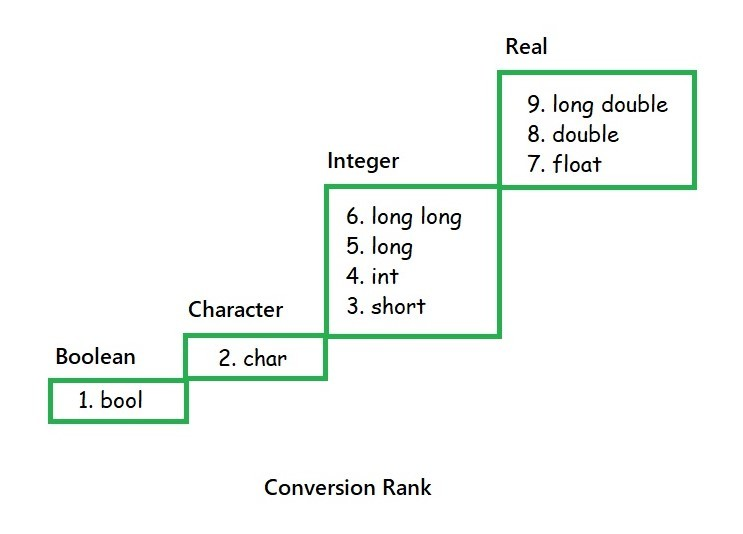# Implicit Type Conversion in C with Examples

• Difficulty Level : Easy
• Last Updated : 25 Nov, 2019

Prerequisite: Data Types, Type Conversion

Implicit Type Conversion is also known as ‘automatic type conversion‘. It is done by the compiler on its own, without any external trigger from the user. It generally takes place when in an expression more than one data type is present. In such condition type conversion (type promotion) takes place to avoid loss of data. All the data types of the variables are upgraded to the data type of the variable with the largest data type.

There are many ways in which the Implicit Type Conversion occurs in C, such as:

• Conversion Rank
A rank can be assigned to the integer and floating-point arithmetic type, from 1 to 9. This scale is theoretically accurate but the actual implementation is not this easy. A long double real has a higher rank than long real and a short integer has a higher rank than a character as shown in the figure.• Conversions in Assignment Expressions
There are two operands and an assignment operator in an assignment operation. The difference in rank decides the promotion and demotion of the right expression to equalize the rank of left and right expression. Promoting implies that the right expression is of lower rank. Demoting implies that the right expression is of higher rank.
• Promotion
Promotion does not create any problems. The rank of right expression is promoted to the rank of left expression. The value of the expression is the value of the right expression after the promotion.

Examples:

```bool        x = true;
char        y = ‘X’;
int         i = 123;
Long double d = 1234.5;

y = x;   // value of y is SOH (ASCII 1)
i = y;   // value of i is 88
d = x;   // value of d is 1.0
d = i;   // value of d is 1234.0

*Here SOH is Start of Header
```
• Demotion
Demotion may or may not create problems. If the size of the variable of the left expression can accommodate the value of the expression, no problem arises but the results can be a bit different from those expected. Any real or integer value can be assigned to the Boolean variable. If the value of the right expression is zero, it implies false(0) is stored. And if the value is something other than zero, i.e. either positive or negative, it implies true(1) is stored.

When an integer or real value is assigned to a character variable, the least significant byte of the number is converted to a character and stored. When a real value is stored in an integer variable only integer part is assigned and the fraction is dropped. But if the integer value is greater than the maximum value that can be stored, then unpredictable results are obtained.

Likewise, if a long double value is stored in a float type variable then the results are valid only if the value fits otherwise very large values become invalid.
Examples:

```bool  b = false;
char  c = ‘X’;
short s = 98;
int   j = INT_MAX;
int   k = 88;

b = c;     // value of b is 1 (true)
s = j;     // value of s is unpredictable
c = k + 1; // demotion: value of c is ‘Y’
```
• Conversions in other Binary Expressions
There are different set of rules for other binary expressions and they become complicated sometimes so summarising them as steps:

1. The operand with the higher rank is determined using the ranking as shown in above figure.
2. The lower-ranked operand is elevated to the rank defined in step 1.
3. The operation is performed with the expression value having the type of the promoted rank.

Examples:

```bool        x = true;
char        y = ‘X’;
int         i = 123;
short       s = 98;
long double d = 1234.5678;

x + y;   // b is promoted, result is ‘Y’ (‘X’ + 1)
i * s;   // result is an int
d * c;   // result is long double
```

Below is an example to demonstrate Implicit Type Conversion of numeric types for a better understanding.

 `// C program to demonstrate Implicit ` `// Type Conversion of numeric types ` ` `  `#include ` `#include ` ` `  `int` `main(``void``) ` `{ ` `    ``// Local Declarations ` `    ``bool` `b = ``true``; ` `    ``char` `c = ``'X'``; ` `    ``float` `d = 1234.5; ` `    ``int` `i = 123; ` `    ``short` `s = 98; ` ` `  `    ``// Statements ` `    ``printf``(``"bool + char is char:     %c\n"``, b + c); ` `    ``printf``(``"int * short is int:      %d\n"``, i * s); ` `    ``printf``(``"float * char is float:   %f\n"``, d * c); ` ` `  `    ``// bool promoted to char ` `    ``c = c + b; ` ` `  `    ``// char promoted to float ` `    ``d = d + c; ` ` `  `    ``b = ``false``; ` ` `  `    ``// float demoted to bool ` `    ``b = -d; ` ` `  `    ``printf``(``"\nAfter execution \n"``); ` `    ``printf``(``"char + true:     %c\n"``, c); ` `    ``printf``(``"float + char:    %f\n"``, d); ` `    ``printf``(``"bool = -float:   %d\n"``, b); ` ` `  `    ``return` `0; ` `} `

Output:

```bool + char is char:     Y
int * short is int:      12054
float * char is float:   108636.000000

After execution
char + true:     Y
float + char:    1323.500000
bool = -float:   1
```

My Personal Notes arrow_drop_up
Recommended Articles
Page :